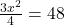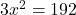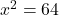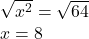Question

Plz I need help with this ASAP

3x^2/4=48

This is solving quadratic functions by square roots btw

1.3x^2 / 4 = 48

Multiply both sides by 4 to get rid of the four on the left.

3x^2 = 192

Divide both sides by 3

x^2 = 64

The square root 64 to get x

x = 8 and x = -8

2.x = 8

Explanation:multiply both sides by 4:divide both sides by 3:take the square root: